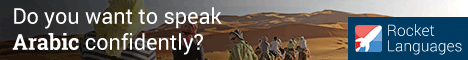- Learn Arabic
 You will learn in this lesson: Arabic articles, definite and indefinite articles.

Learn Arabic اللغة العربية

Arabic Calligraphy

Alphabet (Audio) new!

Vowels

Arabic Phrases (Audio) new!

Articles

Numbers

Pronouns

Feminine & Plural

Verbs

Present Tense

Prepositions

- Body

(new)

(new)

(new)

### Arabic Definite Articles:

The definite article in Arabic is formed by adding the prefix “al” to the noun, “al” is equal to “the” in English and it should be connected to the noun. Examples: the boy = al walad الولد, the girl = al bent البنت , the house = al bait البيت .

The definite article “al, ال ” is not all the time pronounced as “al”, the “al” would be pronounced as “a” instead of “al” if it precedes one of the following letters: (t - ت , th-  ث , d - د , dh- ذ  , r- ر  , z- ز  , s- س  , sh-  ش , s- ص , d -ض  , t - ط  , z- ظ  , n- ن  )

This process is called assimilation. An easy way to make the pronunciation easier, just like the way English does with the indefinite article “a” (a book, but an eagle).

The sky = assamaa السماء (instead of alsamaa) because sky =samaa سماء starts with a (s س  which is part of the letters above).

The moon = alqamar القمر , since the letter q ق  is not in the list above we can always use the full “al” with it.

Note that the omission of the letter “l” is only in pronunciation and not in writing.

Examples:

 Arabic Definite Articles When the “l” is omitted (assimilation) When the “l” is not omitted (no assimilation) The merchant = attajer التاجر (merchant = tajer تاجر ) The notebook = addaftar الدفتر (notebook = daftar  دفتر) The painting = arrasm الرسم (painting = rasm رسم) The time = azzaman الزمن  (time = zaman زمن) The eagle = annasr النسر  (eagle = nasr نسر ) These are just examples of words starting with the letters below: (t - ت , th-  ث , d - د , dh- ذ  , r- ر  , z- ز  , s- س  , sh-  ش , s- ص , d -ض  , t - ط  , z- ظ  , n- ن  ) The moon = alqamar  القمر (moon = qamar قمر ) The sunset = alghuroub  الغروب(sunset =ghuroub غروب ) The eye = al’ayn  العين(eye = ‘ayn عين ) The king = almalik  الملك(king = malik ملك ) The present = alhadiah  الهدية(present = hadiah هدية ) These are just examples of the rest of alphabets that no assimilation is needed for, so you can use the “al” without omitting the “l”. the letters the assimilation is not needed for are: (a - أ , b ب , j ج , h ح ,  kh خ , ‘ ع  , gh غ , f ف  ,q ق , k ك , l ل , m م , n ن ,  h هـ , w  و, yaي  )

Note that whenever an assimilation is needed you need to double the first consonant coming after the first “a” in the word, to understand it more look at the table above.### Arabic Indefinite Articles:

To use the indefinite article in Arabic, there is not much that you can add, just leave the word with no article, especially when you write in Arabic alphabet, there is a small modification however that occurs to the tail of the word, called nunation (adding the suffix “un”):

A house = bait +un = baitun  بيتٌ, the “un” is expressed by this symbol ( ُُ ُُ   or  ٌٌٌٌ  ) when written in Arabic alphabet, like in the example of: baitun = بيتٌ  .

A sun = shams+un = Shamsun شمسٌ ,  a dog = kalbunكلبٌ ,  a tree = shajaratun شجرةٌ , a student = tilmeedun تلميذٌ , a language = lugatun لغةٌ .

Since the nunation is a property of the indefinite article you cannot apply it to the definite article,

The star = annajm النجم , a star = najmun نجمٌ .

 If you are looking for a more extensive Arabic course, we recommend Breaking The Arabic Code